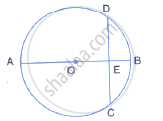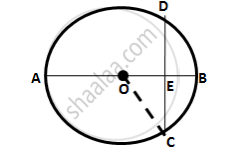Share

# The figure given below, shows a circle with centre O in which diameter AB bisects the chord CD at point E. If CE = ED = 8 cm and EB = 4cm, find the radius of the circle. - Mathematics

Course

#### Question

The figure given below, shows a circle with centre O in which diameter AB bisects the chord CD at point E. If CE = ED = 8 cm and EB = 4cm, find the radius of the circle.#### SolutionLet the radius of the circle be r cm.
∴ OE = OB- EB = r-4
Join OC
In right ΔOEC,
OC^2= OE^2+ CE^2
⇒ r ^2= (r-4)^2+(8)^2
⇒  r ^2 = r ^2-8r +16+64
⇒ 8r = 80
∴ r =10 cm
Hence, radius of the circle is 10 cm.

Is there an error in this question or solution?